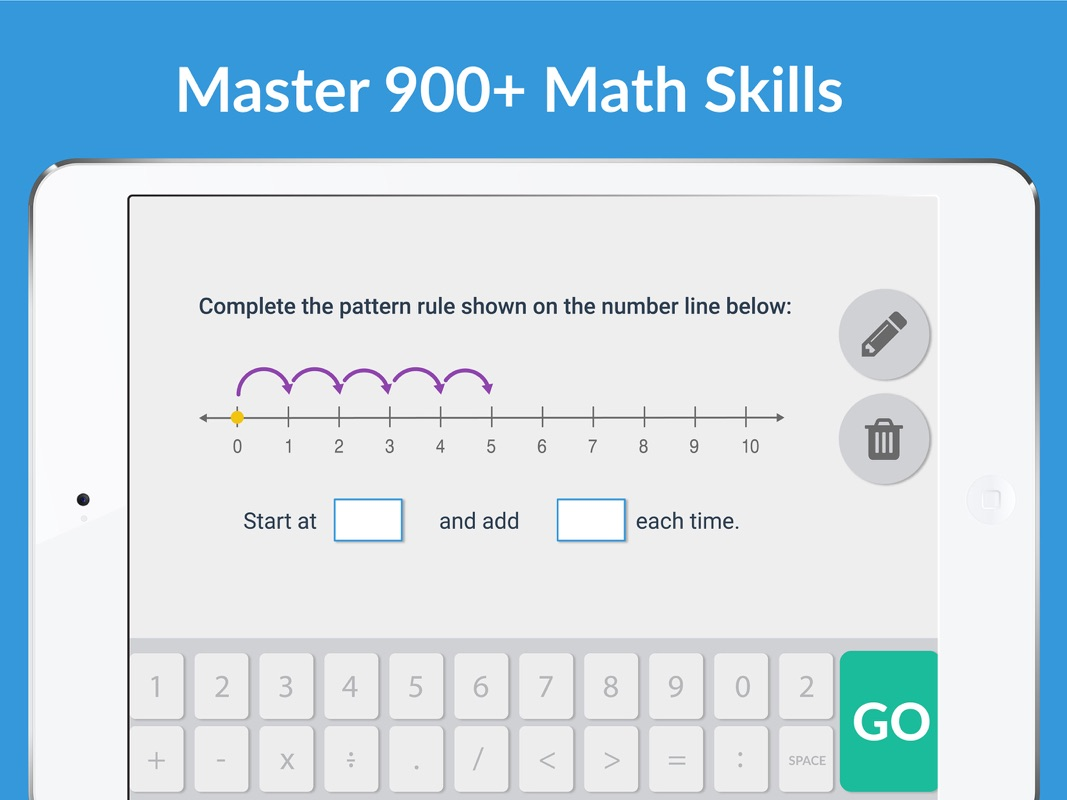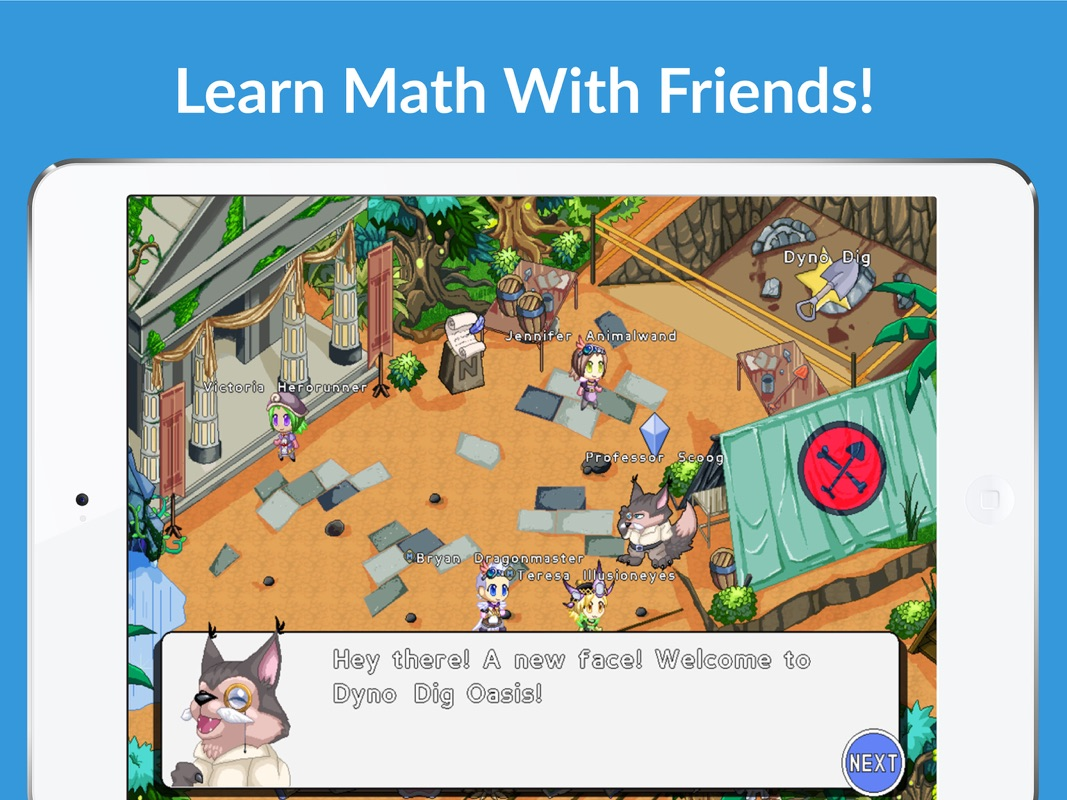## Prodigy Math GameJoin over 15 million teachers and students already using Prodigy to learn math for free! With over 900 crucial math skills for Grades 1 – 8, Prodigy is the perfect game for mastering mathematics both at home and at school.

All educational content in Prodigy is free, and will stay free forever! We only make money through a completely optional game upgrade, which only unlocks some extra content.

Aside from being the world’s most engaging math game, Prodigy also has these great features, all of which are available for free:
1. Full alignment with key curricula (e.g., Common Core, Ontario, TEKS, MAFS)
2. Automatic assessment in-game for all students to place them in the correct grade
4. Embedded in-game formative, diagnostic, and summative assessments.

Each grade covers key mathematics topics that all students must master:
Grade 1 math: comparing numbers, addition to 20, counting, mixed operations, composing numbers, subtraction to 20, 2D shapes, data relationships, place value, addition to 100, ordering numbers, reading numbers, fractions, time, rounding, patterning, subtraction to 100.

Grade 2 math: data relationships, counting, subtraction to 100, addition to 1000, mixed operations, addition to 100, money, subtraction to 1000, 2D shapes, reading numbers, addition to 20, place value, subtraction to 20, comparing numbers, composing numbers, conversion, time, rounding, geometry.

Grade 3 math: multiplication, patterning, division, data relationships, fractions: equivalent, multiplication facts, mixed operations, fractions: represent, 2D shapes, fractions: comparing, division facts, measurement, subtraction to 1000, addition to 1000, rounding, conversion, time, area.

Grade 4 math: fractions: addition and subtraction, patterning, conversion, place value, mixed operations, division, addition to 1000, multiplication, rounding, measurement, fractions: equivalent, subtraction to 1000, data relationships, 2D shapes, fractions: comparing, fractions and decimals, decimals: comparing, factors, addition to 1000000, multiplying fractions, adding to 100, subtracting to 100, money, reading numbers, subtraction to 1,000,000, representing fractions, angles, representing decimals, time.

Grade 5 math: unit conversion, adding and subtracting fractions, multiplication, multiplying fractions, data relationships, dividing fractions, multiplying decimals, pattern rules, adding decimals, 3-digit division, 2d shapes: sides vertices and angles, decimals: place value, mixed operations, comparing fractions, comparing decimals, division: decimals, decimals: mixed operations, numerical expressions, 3D shapes: volume, place value, measurement, representing decimals, expressions and equations.

Grade 6 math: proportional relationships, expressions and equations, 3D shapes, adding decimals, variables, expressions, and equations, geometry, division, subtracting decimals to thousandths, ratios, dividing fractions, reciprocals, comparing rational numbers, evaluating exponents, data relationships, multiplying decimals to thousandths, unit rates, exponent rules, factors and multiples, dividing decimal numbers to tenths, mixed operations with decimals, addition, multiplication, measurement.

Grade 7 math: proportional relationships, probability, multiplying and dividing rational numbers, ratios, data relationships, adding and subtracting rational numbers, variables, expressions, and equations, unit rates, 2D shapes, linear expressions, representing rational numbers, geometry, 3D shapes, rational numbers: mixed operations, integers

Grade 8 math: geometry, linear expressions, expressions and equations, functions, evaluating exponents, exponent rules, scientific notation, data relationships, Pythagorean theorem, irrational numbers, unit rates, angles, statistics, 3D shapes, radicals, variables, expressions, and equations.

For a complete breakdown of all 900+ skills, please visit prodigygame.com/math/skills.

### In-App Purchases

#### Resources

• FREE Prodigy Membership
• FREE Prodigy Membership